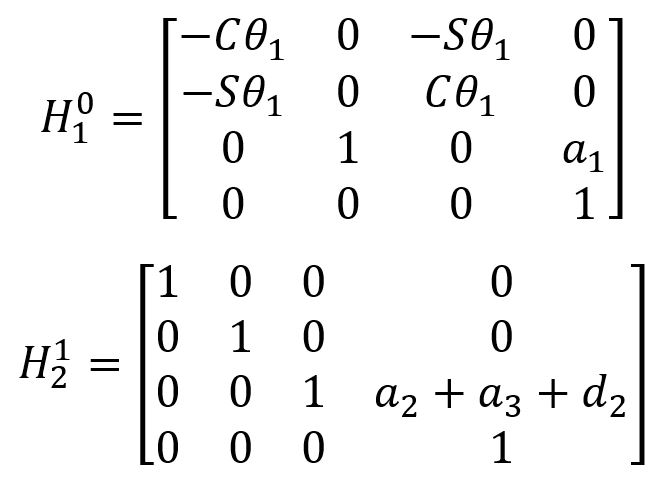Robotics 2
Checkpoint 1: KinematicsExample Question 2:  Shown here is a kinematic diagram that I have labeled, and all of the homogeneous transformation matrices for the manipulator.  Find the Jacobian matrix.
Example Question 3:  Shown here is a Jacobian matrix which I have found.  Do some analysis of the velocities of this manipulator: which velocities will always be zero?  Which velocities are controlled by only one joint?
Example Question 4:  I have decided that I want the end-effector to travel at 2 cm/s in an arc of a sine wave between two points, so I found the parametric equations for a sine wave as shown here.  Find the velocity components of the end-effector.
Example Question 5: I have jumbled up the lines of code to control the path of the end-effector using velocity control.  Put these lines of code in the correct order.
Example Question 7: I have a manipulator that consists of a standard 'arm' and a spherical wrist.  I've already figured out what I want the R0_6 matrix to be, and I've already calculated R0_3 and its inverse, given here.  I've also figured out the R3_6 matrix symbolically, as shown.  Solve for the joint variables Theta 4, Theta 5, and Theta 6.  Then, confirm that your answer is correct by checking the R3_6 matrix.
Example Question 8:  In order to do inverse kinematics on a manipulator with more than 3 degrees of freedom, we have to make an important assumption.  What is that assumption?
Example Question 1: Shown here is a kinematic diagram with no frames drawn in.  Draw in the frames according to the Denavit-Hartenberg rules, label all the link lengths and joint variables, then create a Denavit-Hartenberg table for your drawing.
Example Question 9: Draw a kinematic diagram of a spherical wrist.
Example Question 10:  Shown here is a diagram of a parallel manipulator when the platform is not rotated at all.  Solve for the leg vectors, s1 and s2, when the platform is rotated 30 degrees.
Congratulations!  You've made it all the way through our first unit: Kinematics.  Before we move on, you will need to take a test to make sure that all this information has sunk in.  Here are some example problems like the ones you will see on the test in class.  On our review day (the class day before the test), I'll go through these problems live on the board.  So, it's a good idea to try to solve the problems on your own before coming to class.Example Question 6:  Shown here are six rotation matrices.  Some are valid, and some are invalid.  Circle the matrices you think are invalid, and for each one give a reason as to why you think it is invalid.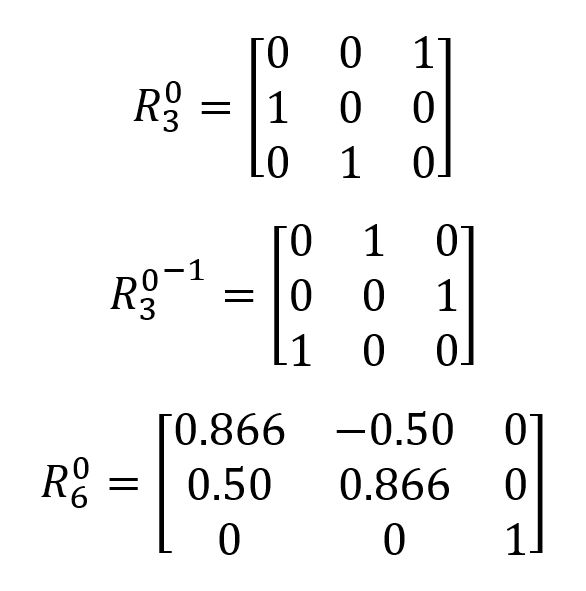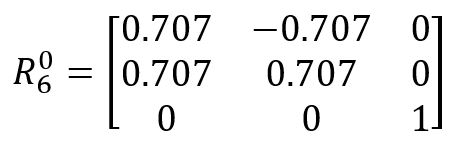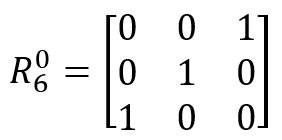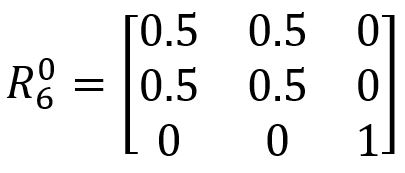Send servos to starting position
Compute Jacobian matrix values
Compute Inverse Jacobian matrix values
Set desired Xdot and Ydot values
Compute joint variable velocities
Command joints to move at computed velocities
Begin loop
End loop